Plotting Spherical Functions edit page

In this chapter various ways of plotting spherical functions are explained. We start by defining some example functions.

## Smooth Plot

The default plot command generates a colored plot without contours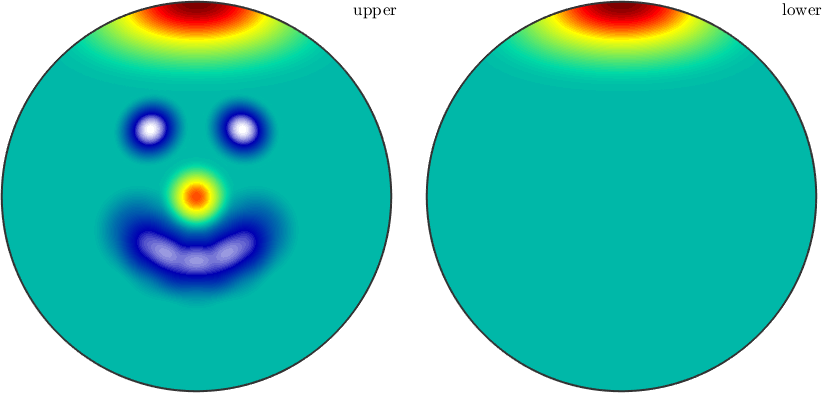• plot(sF1) is the same as pcolor(sF1)

## Contour Plot

nonfilled contour plot plots only the contour lines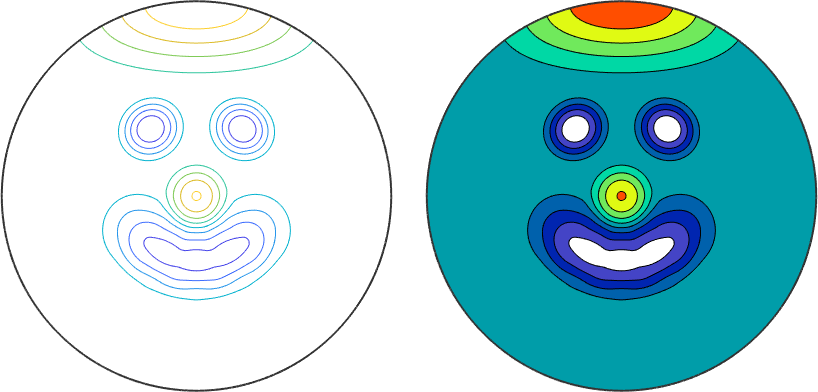## Filled Contour Plot

filled contour plot plots the contour lines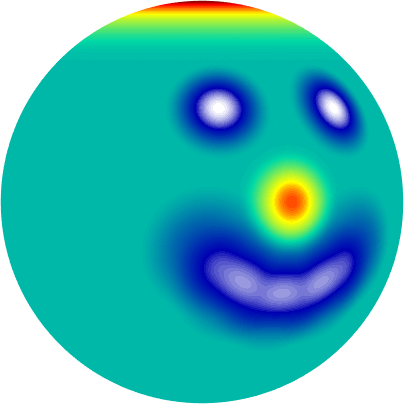## 3D Plot

3D plot of a sphere colored accordingly to the function values.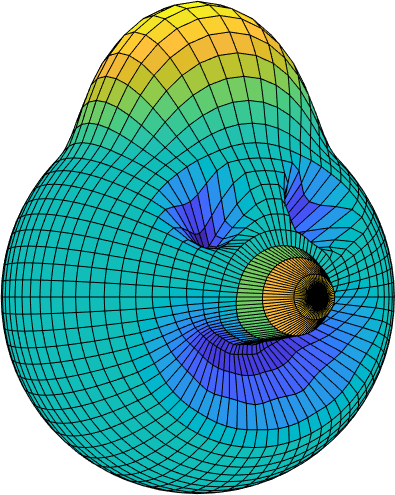## Surface Plot

3D plot where the radius of the sphere is transformed according to the function values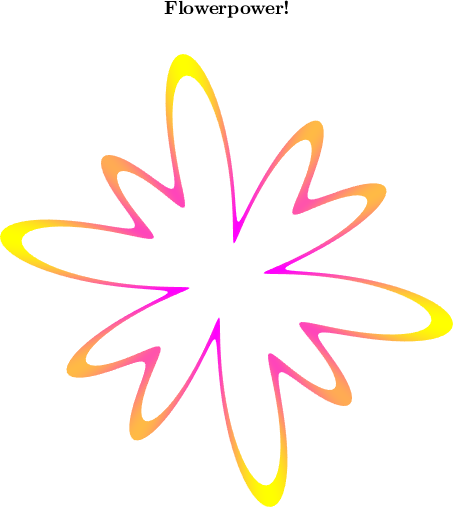## Section Plot

Plot the intersection of the surf plot with a plane defined by a normal vector v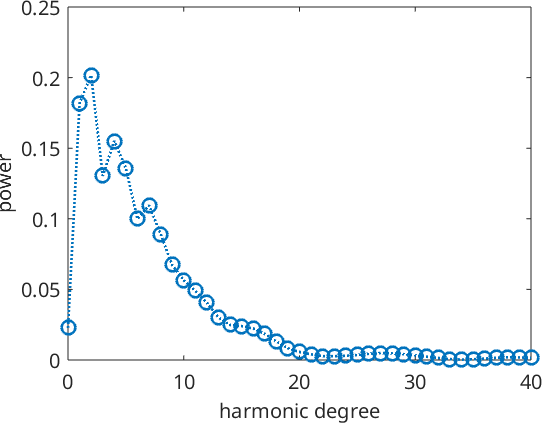## Spectral Plot

plotting the Fourier coefficients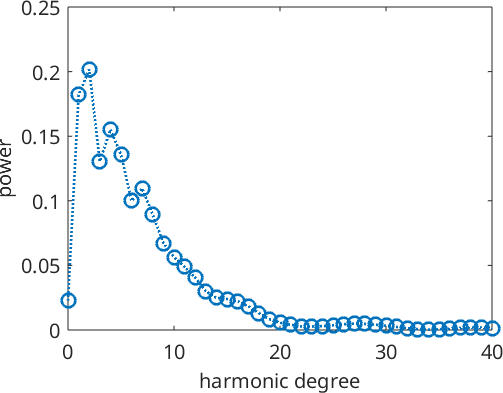The more specific plot options are covered in the respective classes.